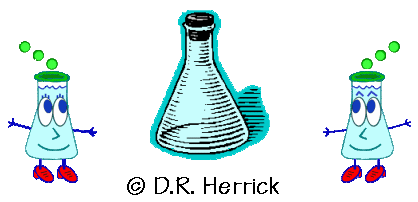Dr. Herrick's CH222 Pressure Quiz 2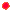Lewis Formulas Copyright D. Herrick20 Questions from past exams. Practice for speed.  Aim for 2 minutes per problem.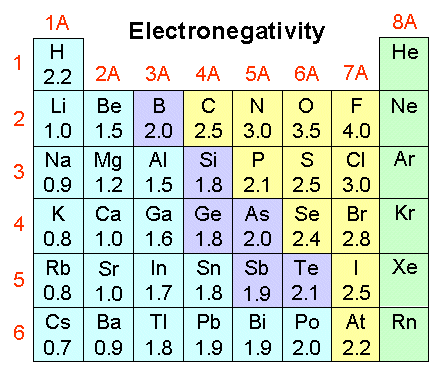1 The Lewis formula for the chlorine molecule shows
 A) a single covalent bond B) a double covalent bond C) a triple covalent bond D) a single ionic bond E) a total of 16 valence electrons
 2 The Lewis formula for the nitrogen molecule shows
 A) a single covalent bond B) a double covalent bond C) a triple covalent bond D) a single ionic bond E) a total of 16 valence electrons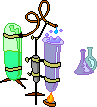3 In the Lewis formula :C:::O: oxygen has
 A) oxidation number = +2, formal charge = +1 B) oxidation number = +2, formal charge = -1 C) oxidation number = -2, formal charge = 0 D) oxidation number = -2, formal charge = +1 E) oxidation number = -2, formal charge = -1
 4 In the Lewis formula :C:::O: carbon has
 A) oxidation number = -2, formal charge = -1 B) oxidation number = -2, formal charge = +1 C) oxidation number = +2, formal charge = 0 D) oxidation number = +2, formal charge = +1 E) oxidation number = +2, formal charge = -1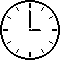5.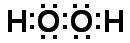In the Lewis formula  oxygen has

 A) oxidation number = -2, formal charge = -2 B) oxidation number = -2, formal charge =  0 C) oxidation number = -1, formal charge = -1 D) oxidation number = -1, formal charge =  0 E) oxidation number = +1, formal charge =  0
 6 The Lewis formula for CO2 shows
 A) two double bonds B) two resonance bonds with bond order 1.5 each C) two single bonds D) one single bond and one double bond E) one single bond and one triple bond
 7 The bond order of the C-N bond in HCN is
 A)  0 B) 1 C)  1.5 D)  2 E)  3
 8 The average bond order of the bonds in O3 is
 A)  0 B)  1 C)  1.5 D)  2 E)  2.5Complete the Lewis structure and answer questions 9-16:   F    N    O
 9 The total number of valence electrons is
 A)  24 B)  22 C) 20 D) 18 E) 16
 10 The bond order between N and O is:
 A)  1 B)  1.5 C)   2 D)   2.5 E)  3
 11 The number of lone pairs of valence electrons on N is
 A)  0 B)  1 C)   2 D)   3 E)  4
 12 The bond order between N and F is
 A)  1 B)  1.5 C)  2 D)  2.5 E)  3
 13 The number of lone pairs of valence electrons on O is
 A)  0 B)  1 C)  2 D)  3 E)  4
 14 The formal charge on O is
 A)  +2 B)  -2 C)  +1 D)  -1 E)  0
 15 The formal charge on N is
 A)  0 B)  -1 C)  +1 D)  -2 E)  +2
 16 The oxidation number on N is
 A)  +1 B)  -1 C)  0 D)  +3 E)  -317.

Which of these violate the octet rule?

 1)  SF2 2)  [AlF6]3- 3)  PCl5
 A) 1 and 3 only B) 2 and 3 only C) 1 and 2 only D) 2 only E) 1, 2, and 3
 18 The correct order of increasing carbon-carbon bond lengths is
 A) H3CCH3  <  H2CCH2  <  HCCH B) H3CCH3  <  HCCH  <  H2CCH2 C) H2CCH2  <  H3CCH3  <  HCCH D) HCCH  <  H2CCH2  <  H3CCH3 E) H2CCH2 <  HCCH  <  H3CCH3
19.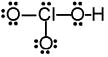The formal charge at chlorine in this Lewis formula is

 A)  +1 B)  +2 C)  0 D)  +3 E)  -1
 20 The number of lone pairs of electrons on the central N atom in nitryl chloride, NO2Cl, is
 A)  0 B)  1 C)  2 D)  3 E)  4